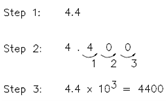## Converting Scientific Notation to Integers Assignment Help

Assignment Help: >> Scientific Notation - Converting Scientific Notation to Integers

Converting Scientific Notation to Integers:

Often, numbers in scientific notation need to be put in integer form. For convert scientific notation to integers:

Step 1: Write decimal number.

Step 2: Move the decimal the number of places specified by the power of ten: to the right if positive, to the left if negative.  Add zeros if essential.

Step 3:  Rewrite the number in integer form. Example:

Convert 4.4 x 103 to integer form.

Solution: